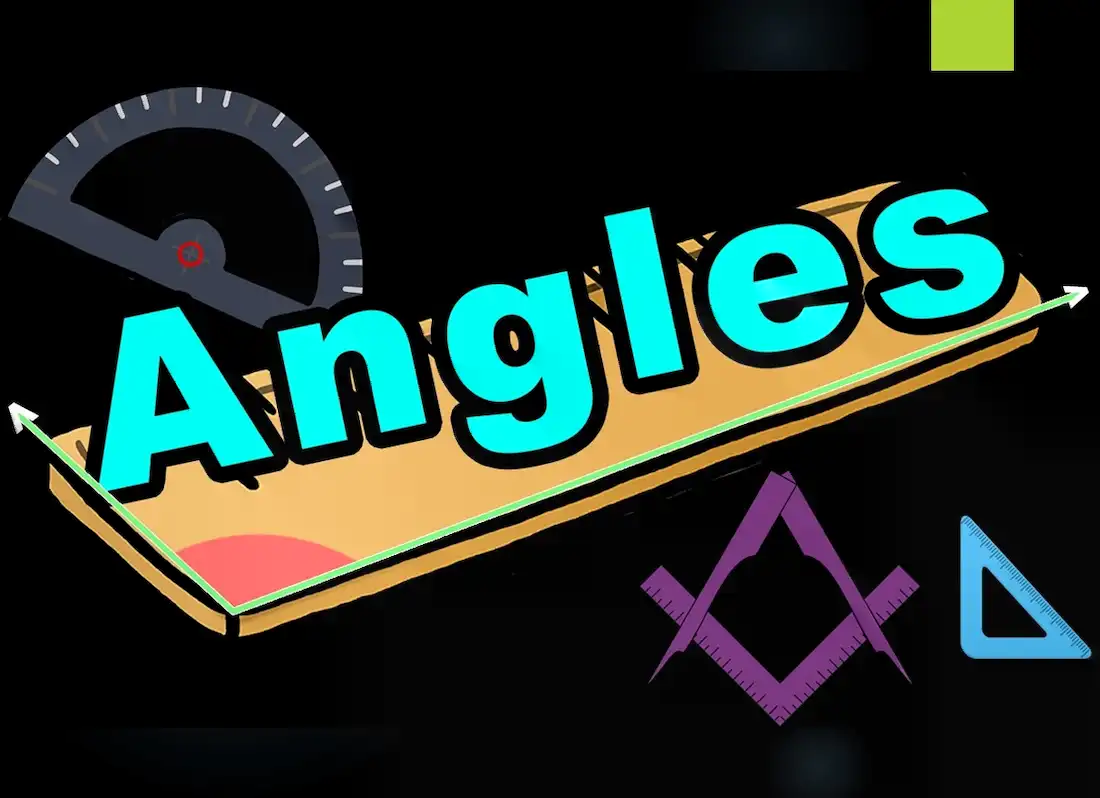Formula Sheets for Geometry Free PDF Download. The geometry formulas are used for finding dimensions, perimeter, area, surface area, volume, etc. of the geometric shapes. Geometry is a part of mathematics that deals with the relationships of points, lines, angles, surfaces, solids measurement, and properties.

There are two types of geometry: 2D or plane geometry and 3D or solid geometry. 2D shapes are flat shapes that have only two dimensions, length, and width as in squares, circles, and triangles, etc. 3D objects are solid objects, that have three dimensions, length, width, and height or depth, as in a cube, cuboid, sphere, cylinder, cone, Let us learn geometry formulas along with a few solved examples in the upcoming sections.

What Are Geometry Formulas?

Formula Sheets for Geometry – The formulas used for finding dimensions, perimeter, area, surface area, volume, etc. of 2D and 3D geometric shapes are known as geometry formulas. 2D shapes consist of flat shapes like squares, circles, and triangles, etc., and cube, cuboid, sphere, cylinder, cone, etc are some examples of 3D shapes. The basic geometry formulas are given as:

List of Geometry Formulas

Below is the list of various geometry formulas for you according to the geometric shape.

• Perimeter of a Square = 4(Side)
• Perimeter of a Rectangle = 2(Length + Breadth)
• Area of a Square = Side2
• Area of a Rectangle = Length × Breadth
• Area of a Triangle = ½ × base × height
• Area of a Trapezoid = ½ × (base1+base2)(base1+base2) × height

Basic geometry formulas where the mathematical constant π is used are,

• Area of a Circle = A = π×r2
• Circumference of a Circle = 2πr
• The curved surface area of a Cylinder  = 2πrh
• Total surface area of a Cylinder = 2πr(r + h)
• Volume of a Cylinder = V = πr2h
• The curved surface area of a cone =  πrl
• Total surface area of a cone = πr(r+l) = πr[r+√(h2+r2)]
• Volume of a Cone = V = ⅓×πr2h
• Surface Area of a Sphere = S = 4πr2
• Volume of a Sphere = V = 4/3×πr3

where,

• h = Height. and,
• l = Slant height

The formula table depicts the geometry formulas used for different 2-D and 3-D shapes:

SHAPES        FORMULAS

• 1. Right Triangle         Pythagoras Theorem: a2 + b2 = c2
• Area = ½ ab
• Perimeter = a + b + √(a2 + b2)

Where,

• c = hypotenuse of a triangle
• a = altitude of a triangle
• b = base of a triangle
• 2. Triangle       Perimeter, P = a + b + c
• Area, A = ½ bh
• Height, h = 2(A/b)

Where,

• a,b,c are the sides of a triangle.
• 3. Rectangle
• Perimeter = 2(l + w)
• Area = lw
• Diagonal, d = √(l2 + w2)

Where,

• l = length of a rectangle
• w = width of a rectangle
• 4.Parallelogram
• Perimeter, P = 2(a + b)
• Area, A = bh
• Height, h = A/b
• Base, b = A/h

Where,

• a and b are the sides of a parallelogram
• h = height of a parallelogram
• 5. Trapezium
• Area, A = ½(a + b)h
• Height, h = 2A/(a + b)
• Base, b = 2(A/h) – a

Where,

• a and b are the parallel sides
• h = distance between two parallel sides
• 6. Circle           Circumference = 2πr
• Area = πr2
• Diameter = 2r

Where,

• r = radius of a circle
• 7. Square         Perimeter, P = 4a
• Area, A = a2
• Diagonal, d = a√2
• Side, a = √A = d/2√2

Where,

• a = side of a square
• 8. Arc  Arc Length, L = rθ
• Area, A = ½r2θ
• Here, θ is the central angle is radians.

Where,

• 9. Cube            Area, A = 6a2
• Volume, V = a3
• Edge, a = V⅓
• Space diagonal = a√3

Where,

• a = side of a cube
• 10. Cuboid      Surface Area, A = 2(lb + bh + hl)
• Volume, V = lbh
• Space diagonal, d = √( l2 + b2 +h2)

Where,

• l= length
• b= breath
• h= height
• 11. Cylinder    Total Surface Area, A = 2πrh + 2πr2
• Curved Surface Area, Ac = 2πrh
• Volume, V = πr2h
• Base Area, Ab = πr2

Where,

• r= radius of a cylinder
• h= height of a cylinder
• 12. Cone          Total Surface Area, A = πr(r+l) = πr[r+√(h2+r2)]
• Curved Surface Area, Ac = πrl
• Volume, V = ⅓πr2h
• Slant Height, l = √(h2+r2)
• Base Area, Ab = πr2

Where,

• r= radius of a cone
• h= height of a cone
• l = slant height
• 13. Sphere       Surface Area, A = 4πr2
• Volume, V = ⁴⁄₃πr3
• Diameter = 2r

Where,

FAQ’s on Geometry FormulasFormula Sheets for Geometry